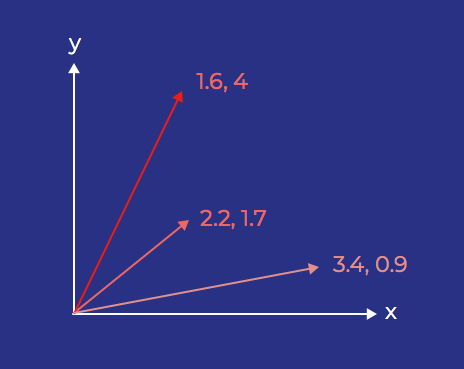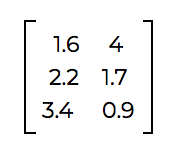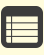Time Estimate

# Apply Deep Learning to Natural Language Processing

## Learning Objectives

After completing this unit, you’ll be able to:
• Explain how a word vector represents word meaning.
• Describe how to visualize word vectors.
• Recall the basic optimization process for a deep learning model.

## Represent Words for Natural Language Processing

Before we can build a model and use deep learning for natural language processing, we have to figure out how to represent words for a computer. In day-to-day life, we represent words in several ways, usually as written symbols (words in text) or as specific sounds (spoken words). Neither of these conveys much to a computer, so we need to take a different approach.

A common solution in machine learning is to represent the meaning of each word as a vector of real numbers.

### Vector Refresher

A vector is a quantity with more than one element. When you’re first working with vectors, it’s easiest to think about two-dimensional vectors because we’re very comfortable working in two-dimensional space. For these vectors, we usually think of their two elements as representing magnitude (how long they are) and direction (which way they extend from their origin). We can visualize these vectors by plotting them as arrows on a chart, starting from the origin and extending to different points on the plane.You can also represent a set of vectors as a matrix where each row represents an individual vector.In reality, the vectors we use to represent words have far more than two dimensions. Many word vectors use 300 dimensions. It’s hard to imagine what a vector in 300-dimensional space looks like, but the idea (and the math!) is very similar to a two-dimensional vector. We can visualize these high-dimensional vectors by reducing their dimensionality and plotting them in two dimensions. We can also represent them as matrices the way we did above.

You can think of each number in a word’s vector as a feature. We use deep learning to create these vectors and "choose" the features. Remember, because machines design these vectors through deep learning, they’re not easily described, like "is an animal" or "is a verb." Each number represents a feature determined by the model itself.

We use these word vectors to plot words in a high-dimensional vector space. When we plot word vectors this way, words with similar meanings tend to cluster together.

So for example, in a given word cloud, all the names of countries might cluster together, because they are similar. Nearby, you might find words like state, national, and international. Because human beings have a hard time imagining multidimensional spaces, we use dimensionality reduction to project word vectors down to two or three dimensions for visualizations.#### Note

What are the axes for this kind of word plot? Essentially, nothing in particular. Remember, we’re looking at a 300-dimensional space, squished down to two dimensions, so we’ve combined many features to make these two axes. Sometimes, there may seem to be axes in a word cloud, like increasing intensity of adjectives in one direction, or increased specificity of nouns, but the axes aren’t actually that simple. Don’t worry too much about figuring out what any specific axis means.

## Loss Functions and Optimization

Once you have represented words using vectors, the next thing you need to train your model is a loss function (also sometimes called an objective function).

Because no model is perfect, you should expect any deep learning model to have some level of error. A loss function is essentially a distance function between the expected value for a given decision and the actual value the model comes up with. The loss function describes your model’s error.

As you train your model, you are trying to minimize this loss function, bringing the model’s decisions as close as possible to your expected results. A toy example, like the one Richard shared in his video, might have a loss function as simple as adding a uniform value to the model’s error for every incorrect decision it makes. Most real-world applications use more complex loss functions.

For example, mean squared error (MSE) is a popular loss function. Mean squared error is the average squared difference between the model’s values and the actual expected values. If you've studied any introductory machine learning materials, you may have come across MSE using this equation.#### Note

Note: For a refresher on how you can derive that equation, check out Khan Academy’s video, Squared error of regression line.

One common application for MSE is as the loss function for a k-nearest neighbors classifier.

To optimize a model, you need both a loss function (to measure your current model’s error) and an optimizer (to make changes to the model in an effort to reduce that measurement of error).

The optimizer helps the model "learn" how to make good decisions by changing the model’s parameters. The goal of modifying these parameters is to get a model that results in less error. Another way to look at this is that we want our optimizer to minimize the loss function.

## Get Hands On with Natural Language Processing

In this trail, you’ll complete problem sets using a Google product called Colaboratory. That means you have to have a Google account to complete the challenges. If you don’t have a Google account or you want to use a separate one, you can create an account here.

Once you have a Google account:
3. Go to Colaboratory.
5. Choose the source code file (.ipynb) and click Open.

Now you’re ready to start coding! Each piece of code is contained in cells. When you click into a cell, a play button appears. This button lets you run the code in the cell.

Throughout the worksheet, you’ll find exercise markers that let you know you need to do something. After you complete an exercise, come back to Trailhead to answer the corresponding question about your results. Note that you may get an error message that your versions of torchvision and fastai are incompatible. This is OK and will not stop you from getting the right results. Forge ahead.#### Note

Before you run a cell, make sure you run all the cells above it first.

Have fun!